# Diffy naive

A week or so ago Rob Eastaway posted about the game Diffy on Twitter. Diffy begins with four numbers arranged around a cycle. Taking the (absolute values of) differences between adjacent pairs produces four more numbers around a cycle. If you start with positive integers then iterating this process eventually reaches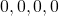. How many iterations does it take?

A quick search (and the existence of Rob Eastaway’s talk on the subject) reveals that a fair amount is known about Diffy. I have deliberately not read any of it in detail. I don’t even know why you always reach zero, which is not as obvious as I had assumed: consider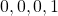, say, which reaches zero in four steps, but increases the sum of the four numbers along the way. The question I looked at was “What is the maximum number of steps this process can take, beginning with numbers from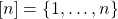?” Call this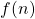.

Rob begins by asking us to show that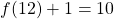. (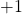by counting the cycles we see along the way rather than the number of steps we take.) There are only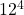starting points so this takes no time at all if we break the spirit of the puzzle and use a computer. But there’s no need to be pointlessly inefficient. The length of a Diffy “game” is unchanged if we

• add a constant to every number
• rotate the numbers around the cycle
• reverse the order of the numbers around the cycle
• (multiply the numbers by a non-zero constant)

By applying the first three observations we can say that there will be a witness for the value ofusing numbers from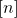with the first number being, and the fourth number being at least as large as the second.

f' [x,y,z,w] = [abs (x-y), abs (y-z), abs (z-w), abs (w-x)]

g' xs = takeWhile (/=[0,0,0,0]) (iterate f' xs)

h' xs = length (g' xs) + 1

i' n = maximum [(h' [a,b,c,d], [a,b,c,d]) | a <- , b <- [a..n], c <- [a..n], d <- [b..n]] 

(Not pictured: good choice of variable names.)

So an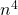exhaust is down to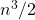using symmetry.

My next thought in these situations is that we’re doing an awful lot of recomputation. If I’ve already scored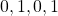, why should I reevaluate it when scoring? Why not store the score somewhere so we can look it up later?

f n = maximum (elems table) where
table = array ((0,0,0),(n-1,n-1,n-1)) [((a,b,c), g a b c) | a <- [0..n-1], b <- [0..n-1], c <- [0..n-1]]
g 0 0 0 = 1 -- represents c c c c for c > 0 the first time you reach it
g a b c | a > c = g c b a
| otherwise = (table ! normalise a (abs (b-a)) (abs (c-b)) c) + 1

normalise a b c d = let m = minimum [a,b,c,d]
in  normalise2 (a-m) (b-m) (c-m) (d-m)

normalise2 0 b c d = (b,c,d)
normalise2 a 0 c d = (c,d,a)
normalise2 a b 0 d = (d,a,b)
normalise2 a b c 0 = (a,b,c)

This is what I ended up with. It isn’t pretty. The thing that wasn’t, but should have been, immediately obvious is that the states I encounter along the way won’t be in the nicely normalised form we’ve just decided we want to work with, so the naive lookup table increases our time back up to. On the other hand, normalising things like this is expensive compared to the computation we’re trying to save. It’s a very bad deal, and even if it weren’t I can’t afford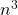memory for very large values of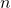.

So we’re back to computinghonestly for eachin time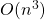. The next observation is that if we wantfor lots ofwe can do better: ifis to be larger than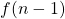then the pattern witnessing that had better use bothand, taking us to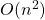for each new. In fact, we can say a bit more. Theandwould either have to be adjacent or opposite.

    1 -- b        1 -- n
|    |        |    |
d -- n        d -- c

Then we still have some symmetries to play with with. In the opposite case, all of the edges are related by symmetries so we can assume that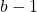is the smallest difference. In the adjacent case there is less symmetry, but we can still assume that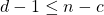.

direct n = maximum ( [score 1 b n d | b <- [1..nquot2], d <- [b..n+1-b]]
++ [score 1 n c d | c <- [1..n], d <- [1..n+1-c]] )

score 0 0 0 0 = 0
score a b c d = 1 + (score (abs (a-b)) (abs  (b-c)) (abs (c-d)) (abs (d-a)))

That’s not bad and will get you well up into the thousands without difficulty.

(Everything above is a mostly accurate representation of my progress through this problem, with light editing to fix errors and wholesale removal of dollar signs from my Haskell, since they confuse the LaTeX plugin. What follows is ahistorical, presenting an endpoint without all the dead ends along the way.)

So far we haven’t thought about the actual operation we’re iterating, so let’s do that now. Suppose that we’re in the opposite case with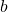and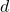strictly betweenand. Then replacingby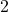andby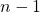produces a pattern that goes to the same place as the original pattern under two rounds of iteration, so such patterns aren’t interesting in our search; they were considered in previous rounds. Similarly, in the adjacent case where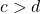we can decrease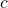andto obtain an equivalent-after-two-iterations pattern with a smaller value of, so we don’t need to consider it. Finally, if we’re in the opposite case but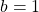, say, then we could alternatively have viewed ourself as being in the adjacent case. So we can reduce our search to

direct n = maximum [score 1 a b n | a <- [1..n], b <- [a..n]]

for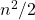or, after further assuming that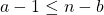,

direct n = maximum [score 1 a b n | a <- [1..(n+1)]quot2], b <- [a..n-a+1]]

for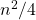. (Once these computations are taking hours or days the constant factor improvements shouldn’t be undervalued.)

We’ve already seen that storing lots of information about previously computed results is not helpful, but we can store the known values ofand its “inverse”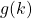, the leastsuch that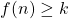. Then when testing whether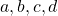scores at least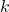it might be worth checking whether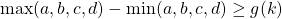, which is the absolute minimum requirement to last at leastrounds. But our scoring function is so cheap that you don’t have to do very much at all of this sort of thing before it becomes too expensive, and in practice the optimal amount of checking seems to be zero.

Unless we can somehow do the checking without actually doing the checking? If we’re currently trying to check whether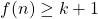then we’d better have the smallest and largest differences differing by at least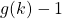. That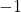is the point at which I throw my hands up and switch to the interval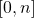rather than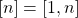, which means you have to keep an eye out for off by one errors when comparing earlier results with what comes next. We already have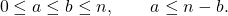The largest difference at the beginning is, so we additionally require that either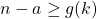or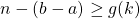. Rearranging, either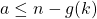or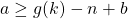. This takes us to

newRecord n = maximum [score 0 a b n | b <- [..n], a <- h b]
where
gk = firstTimeWeCanGet ! (recordUpTo ! (n-1)) -- see full listing
h b = let top = min b (n-b) in
if   n-gk < b-n+gk && n-gk < top
then [0..n-gk] ++ [b-n+gk..top]
else [0..top]


with a cheap improvement from doing the first round of the iteration by hand.

newRecord n = maximum [score a (b-a) (n-b) n | b <- [0..n], a <- f b] + 1

There’s at least one more idea worth considering. We haven’t used the fourth symmetry, of multiplying by non-zero constants. The practical use would be to divide out any common factors of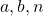, but doing the gcd each time is too expensive. I had greater hopes for checking that at least one of them is odd, which should save a quarter of the work half of the time, for a total saving of about 12%, but it doesn’t seem to help, even if you enforce it by never generating the bad pairs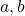, the same way we are able to do for the size consideration in the current listing.

This will produce the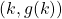pairs up to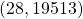in 100 minutes on my 3.6GHz machine. It hasn’t found the next pair yet after a few days of searching. It’s possible that some of the optimisation considerations (especially for what should be the cheap cases like eliminating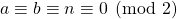) change for largeas naive scoring becomes more expensive, but my haphazard trials have had inconsistent results, both algorithmically and in terms of apparently making the compiler stop favouring certain optimisations.

(0,0)
(1,1)
(2,1)
(3,1)
(4,1)
(5,3)
(6,3)
(7,4)
(8,9)
(9,11)
(10,13)
(11,31)
(12,37)
(13,44)
(14,105)
(15,125)
(16,149)
(17,355)
(18,423)
(19,504)
(20,1201)
(21,1431)
(22,1705)
(23,4063)
(24,4841)
(25,5768)
(26,13745)
(27,16377)
(28,19513)